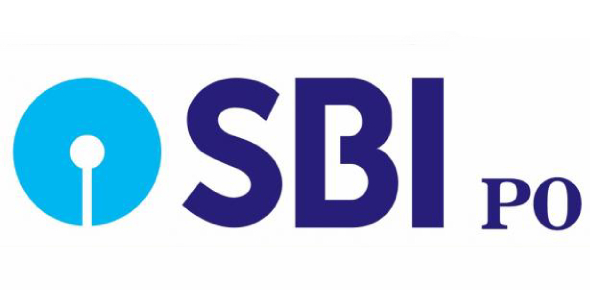SBI PO - Di Quiz -1

5 Questions | Total Attempts: 110SettingsHow much can you remember of the pie chart Mathematics? We all know that in total it adds up to 360. The quiz below tests and jogs your mind on the basics of interpreting a pie chart.

• 1.
What is the value of half of the difference between the number of students in MBA and MBBS?
• A.

650

• B.

800

• C.

1600

• D.

1300

• E.

None of these

• 2.
What is the total number of students in B. Ed., Pharmacy and MBBS together ?
• A.

2465

• B.

2565

• C.

2405

• D.

2504

• E.

None of these

• 3.
How much more percentage (approximate) of students are in MBA as compared to students in B.Ed. ?
• A.

49%

• B.

53%

• C.

59%

• D.

41%

• E.

44%

• 4.
What is the respective ratio between the number of the students in Pharmacy and the number of the students in B.Tech?
• A.

11 : 13

• B.

13 : 6

• C.

13 : 7

• D.

6 :13

• E.

None of these

• 5.
Number of students in B. Sc. is approximately what percentage of the number of students in B. Ed. ?
• A.

167%

• B.

162%

• C.

157%

• D.

153%

• E.

None of these

Related TopicsBack to top